Monday 08th August 2022CBSE Guess > Papers > Important Questions > Class XI > 2010 > Maths > Maths By Mr. Anil Kumar Tondak CBSE CLASS XI
Binomial Theorem Q.1. Find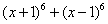. Hence, Evaluate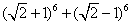. Q.2. Show that 9n+1 – 8n -9 is divisible by 64. Q.3. Find a if the 17th and 18th terms of the expansion of (2 + a)50 are equal. Q.4. Find the middle term in the expansion of (1 + x)2n, where n is a positive integer. Q. 5. Find a positive value of m for which the coefficient of x2 in the expansion of (1 + x )m is 6. Q.6. Find the middle terms in the expansions of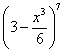. Q.7. Find the term independent of x in the expansion of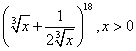Q.8. Find the coefficient of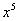in the product (1 + 2x)6 (1 – x)7 using binomial theorem. Q.9. Find the rth term from the end in the expansion of (x + a)n. Q.10. Find the expansion of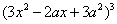using binomial theorem. Q.11. In the expansion of (1+a)m+n, prove that coefficients of am and an are equal. Q.12. The second, third and fourth terms in the binomial expansion of (x + a)n are 240, 720 and 1080 respectively. Find x, a and n. Q.13. If the coefficients of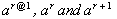in the expansion of the (1 + a)n are in arithmetic progression, prove that n2 - n(4r +1) + 4 r2 – 2 = 0. Q.14. The coefficients of the (r – 1)th , rth and (r + 1)th terms in the expansions of (x +1)n are in the ratio of 1 : 3 : 5. Find n and r. Q.15. Find n if the ratio of the 5 th term from the beginning to the 5 th term from the end in the expansion of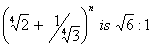. Q.16. Evaluate :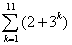. Q.17. Find the value of a so that the term independent of x in the expansion of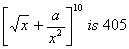Q.18. Find the 11th term from the end in the expansion of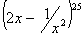Paper By Mr. Anil Kumar Tondak Email Id : [email protected] Ph No.: 9811363962